# python怎么删除列为空的行

python删除列为空的行的方法：首先使用dropna()方法，找到DataFrame类型数据的空值；然后将空值所在的行删除；再将新的DataFrame作为返回值返回即可删除列为空的行。1.摘要

dropna()方法，能够找到DataFrame类型数据的空值（缺失值），将空值所在的行/列删除后，将新的DataFrame作为返回值返回。

2.函数详解

axis：轴。0或’index’，表示按行删除；1或’columns’，表示按列删除。

how：筛选方式。‘any’，表示该行/列只要有一个以上的空值，就删除该行/列；‘all’，表示该行/列全部都为空值，就删除该行/列。

thresh：非空元素最低数量。int型，默认为None。如果该行/列中，非空元素数量小于这个值，就删除该行/列。

subset：子集。列表，元素为行或者列的索引。如果axis=0或者‘index’，subset中元素为列的索引；如果axis=1或者‘column’，subset中元素为行的索引。由subset限制的子区域，是判断是否删除该行/列的条件判断区域。

inplace：是否原地替换。布尔值，默认为False。如果为True，则在原DataFrame上进行操作，返回值为None。

3.示例

```import numpy as np
import pandas as pd

a = np.ones((11,10))
for i in range(len(a)):
a[i,:i] = np.nan

d = pd.DataFrame(data=a)
print(d)```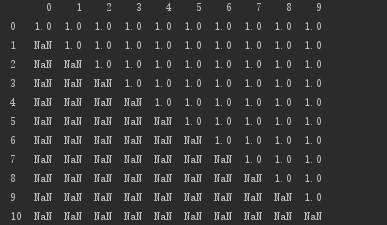```# 按行删除：存在空值，即删除该行
print(d.dropna(axis=0, how='any'))```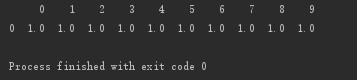```#  按行删除：所有数据都为空值，即删除该行
print(d.dropna(axis=0, how='all'))```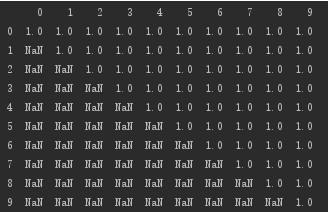```# 按列删除：该列非空元素小于5个的，即删除该列
print(d.dropna(axis='columns', thresh=5))``````# 设置子集：删除第0、5、6、7列都为空的行
print(d.dropna(axis='index', how='all', subset=[0,5,6,7]))``````# 设置子集：删除第5、6、7行存在空值的列
print(d.dropna(axis=1, how='any', subset=[5,6,7]))```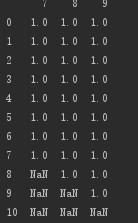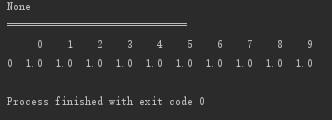```# 原地修改
print(d.dropna(axis=0, how='any', inplace=True))
print("==============================")
print(d)```
Python开发开发项目